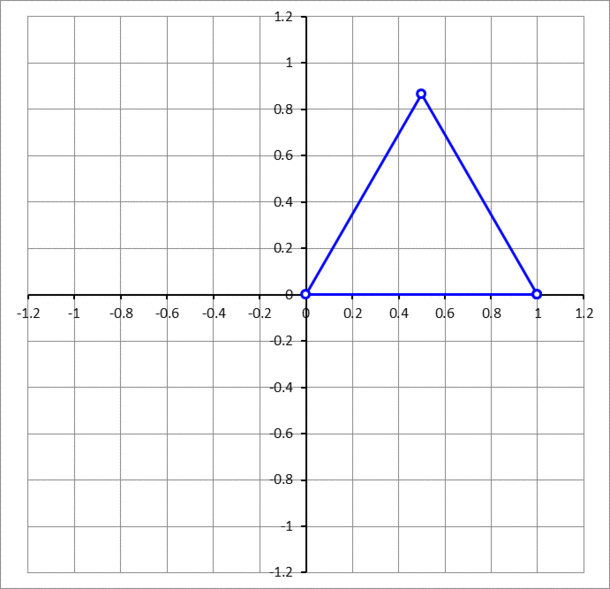# A sliding equilateral triangle

Geometry Level 4A unit equilateral triangle has two vertices sliding on the $x$- and $y$-axis, while the third vertex traces a curve, as shown above. The curve is an ellipse. Find its area.

Inspiration

×